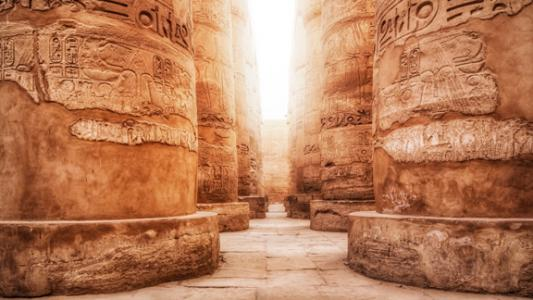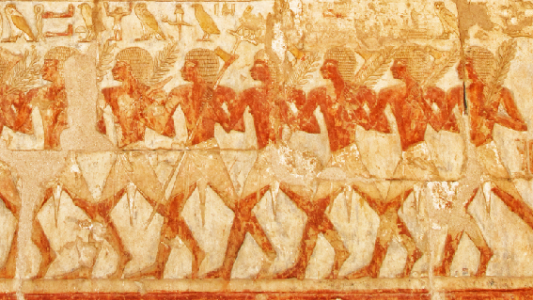# Fractions

The world of fractions is much more interesting than you might imagine! We've collected together activities where you can explore properties of fractions, and make some unexpected discoveries about the world of rational numbers.### Egyptian Fractions

Egyptian fractions are sums of unit fractions. We invite you to explore ways of expressing a fraction as an Egyptian Fraction.### Egyptian Fractions - How Many Ways?

Can you find many different ways to write a fraction as an Egyptian Fraction? And what does it have to do with factors?### Egyptian Fractions - Increasing the Numerator

Once you can write unit fractions as Egyptian Fractions, you might like to try increasing the numerator...### Egyptian Fractions - Writing them all

Explore how using a so-called Greedy Algorithm can help you to express different fractions as Egyptian Fractions. Will the method work for every fraction?